Related Articles

# Bar Graph in MATLAB

• Last Updated : 09 Apr, 2021

A Bar Graph is a diagrammatic representation of non-continuous or discrete variables. It is of 2 types vertical and horizontal. When the height axis is on the y-axis then it is a vertical Bar Graph and when the height axis is on the x-axis then it is a horizontal Bar Graph.

In MATLAB we have a function named bar() which allows us to plot a bar graph.

Syntax:

bar(X,Y)
where X and Y represent the x and the y axis of the plane. The X and Y both are vectors.

Now let’s move to some examples.

Example 1: A simple Bar graph:

## MATLAB

 `% Coordinates of x-axis``x=100:20:160;``% Coordinates of y-axis``y=[22 44 55 66];`` ` `% Bar function to plot the Bar graph``% Set the width of each bar to 60 percent``% of the total space available for each bar``% Set the bar color green``bar(x,y,0.6,``"green"``);`

Output :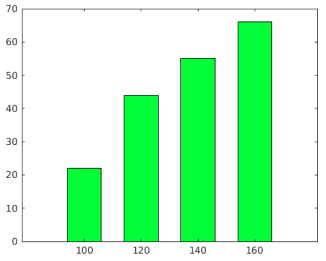Example 2: 3 groups of 4 bars:

## MATLAB

 `% 3 groups are made with 4 bars``% ";" is used to seperate the groups``y=[2 5 4 1; 5 3 3 1; 2 8 4 6];`` ` `% bar function to plot the bar``bar(y);`

Output :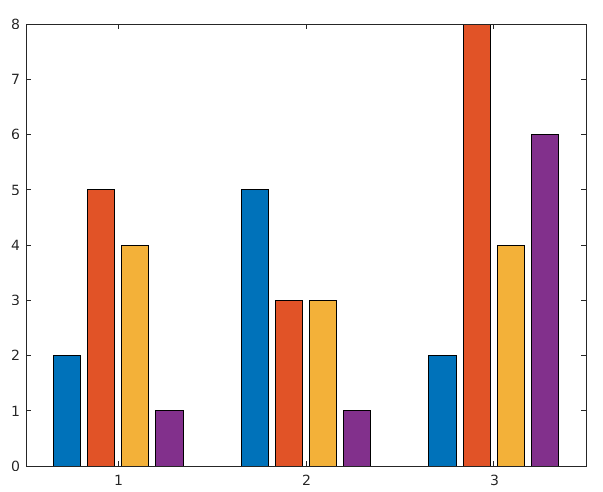Example 3: Display Stacked bars:

## MATLAB

 `% 3 groups``y=[2 5 4 1; 5 3 3 1; 2 8 4 6];`` ` `% stacked is used to stack the bars ``% on each other``bar(y,``'stacked'``);`

Output :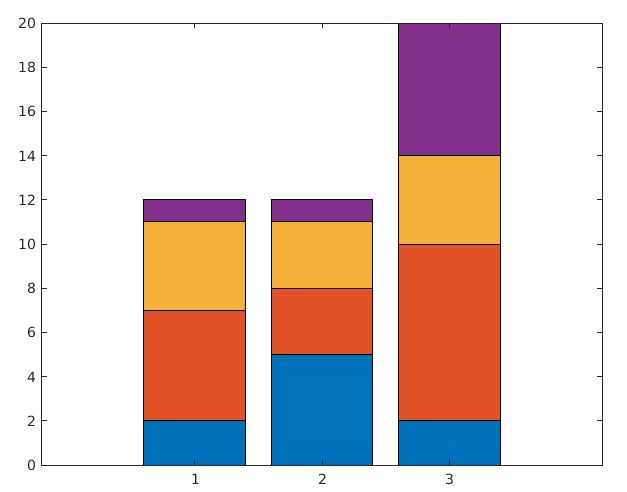Example 4: Display negative bars:

## MATLAB

 `% bars with negative values``y=[2 5 4 -1; 5 -3 3 1; -2 8 4 6];`` ` `% bar function to display bars``bar(y);`

Output :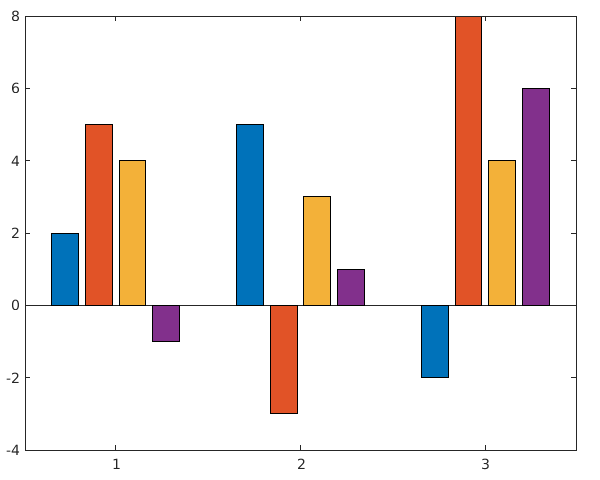Example 5: Display horizontal bar graph:

## MATLAB

 `% Coordinates of y axis``y=[2 5 4 1];`` ` `% barh() function is used to ``% display bar horizontally``barh(y);`

Output :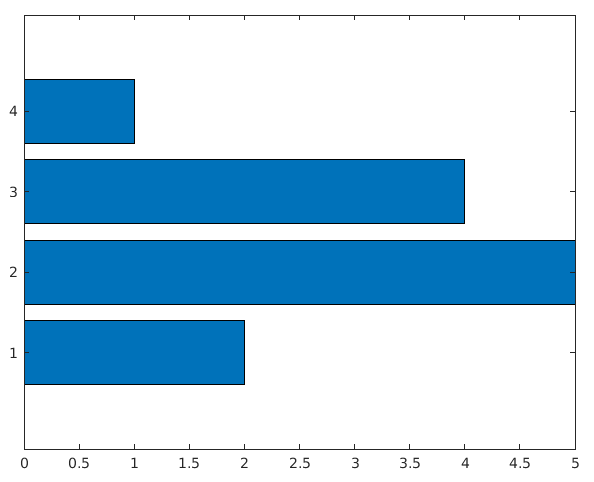My Personal Notes arrow_drop_up